# Graphing a Quadratic Function

Graphing a Quadratic Function

Here is a rocket getting launched and it can go as high as 1000 ft!

It will go up for a few seconds after the engine is ignited.As the engine burns out, the rocket will be in freefall.

Now, Cooper will investigate the time and height attained by the rocket.He describes the path by a quadratic function.

The word "quadratic" is derived from the word "quad" which means square.

In other words, a quadratic function is a “polynomial function of degree 2.”

In this mini-lesson, we will explore the world of graphs of quadratic functions. You will get to learn the domain and range of quadratic function, quadratic graph, vertex form of quadratic function, graphing quadratic functions in vertex form, standard form of a quadratic function, graphing quadratic functions in standard form, quadratic function graph, and other interesting facts around the topic.

You can also check out the playful calculators to know more about the lesson and try your hand at solving a few interesting practice questions at the end of the page.

Let's go ahead and learn more about this topic now.

## Lesson Plan

 1 What Is a Quadratic Function? 2 Tips and Tricks 3 Important Notes 4 Solved Examples 5 Interactive Questions

## What Is a Quadratic Function?

### Quadratic Function: Definition

A function of the form $$f(x)=ax^2+bx+c$$, where $$a \neq 0$$ is called a quadratic function.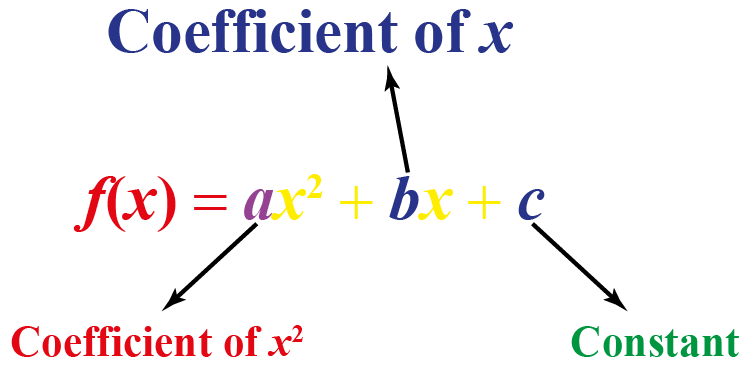### Domain and Range of Quadratic Function

A quadratic function is a polynomial function that is defined for all real numbers.

So, the domain of a quadratic function is the set of real numbers, that is, $$\mathbb{R}$$.

The range of quadratic function depends on the graph's opening side and vertex.

So, look for the lowermost and uppermost $$y$$ values on the graph of the function to determine the range of the quadratic function.

### Standard Form of a Quadratic Function

The standard form of a quadratic function is $$f(x)=ax^2+bx+c$$, where $$a \neq 0$$.

Sometimes, a quadratic function is not written in its standard form, $$f(x)=ax^2+bx+c$$, and we may have to change it into the standard form.

Here are some examples of functions and their standard forms.

 Functions Steps to follow Standard Form $$f(x)$$= $$(x-1)(x+2)$$ Multiply the factors $$(x-1)$$ and $$(x+2)$$ $$f(x)$$= $$x^2+x-2$$ $$f(x)-x^2$$= $$-3x+1$$ Move the term $$-x^2$$ to the right side of the $$=$$ sign $$f(x)$$= $$x^2-3x+1$$ $$f(x)+5x(x+3)$$ =$$12x$$ Expand the bracket on the left side and move $$12x$$ to the left side of the $$=$$ sign $$f(x)$$= $$-5x^2-3x$$ $$f(x)+x^3$$= $$x(x^2+x-3)$$ Expand the bracket on the right side and move $$x^3$$ to right side of the $$=$$ sign $$f(x)$$= $$x^2-3x$$

### Vertex Form of Quadratic Function

The vertex form of quadratic function is $$f(x)=a(x-h)^{2}+k$$, where $$(h,k)$$ is the vertex of the parabola.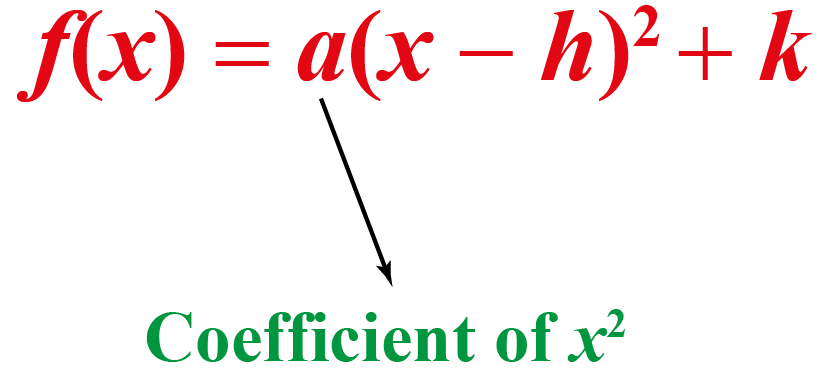This shows the vertex form of a quadratic function.

## How to Graph Quadratic Functions?

### Graphing Quadratic Functions in Vertex Form

Now, in terms of graphing quadratic functions, we will understand a step-by-step procedure to plot the graph of any quadratic function.

Consider the general quadratic function $$f\left( x \right) = a{x^2} + bx + c$$.

First, we rearrange it (by the method of completion of squares) to the following form:

$f\left( x \right) = a{\left( {x + \frac{b}{{2a}}}\right)^2} - \frac{D}{{4a}}$

The term D is the discriminant, given by $$D = {b^2} - 4ac$$.

If you are unsure about this rearrangement, you are urged to refer to the chapter on quadratic equations.

Now, to plot the graph of $$f(x)$$, we start by taking the graph of $${x^2}$$ ,and applying a series of transformations on it:

Step-1: $${x^2} \to a{x^2}$$: This will imply a vertical scaling of the original parabola. If $$a$$ is negative, the parabola will also flip its mouth from the positive to the negative side. The magnitude of the scaling depends upon the magnitude of $$a$$.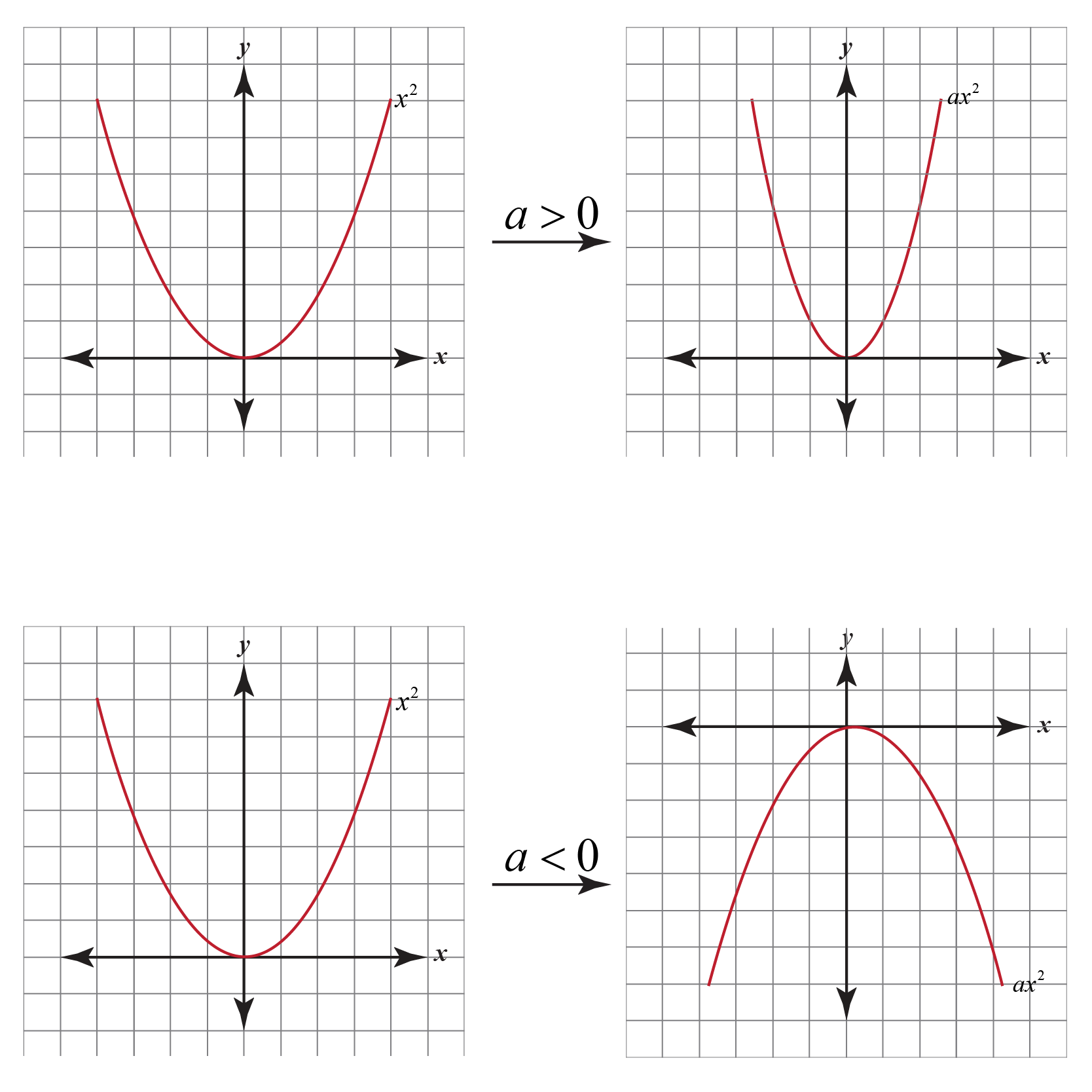Step-2:  $$a{x^2} \to a{\left( {x + \dfrac{b}{{2a}}}\right)^2}$$: This is a horizontal shift of magnitude $$\left| {\dfrac{b}{{2a}}} \right|$$ units. The direction of the shift will be decided by the sign of $$\dfrac{b}{{2a}}$$. The new vertex of the parabola will be at $$\left( { -\dfrac{b}{{2a}},0} \right)$$. The following figure shows an example shift: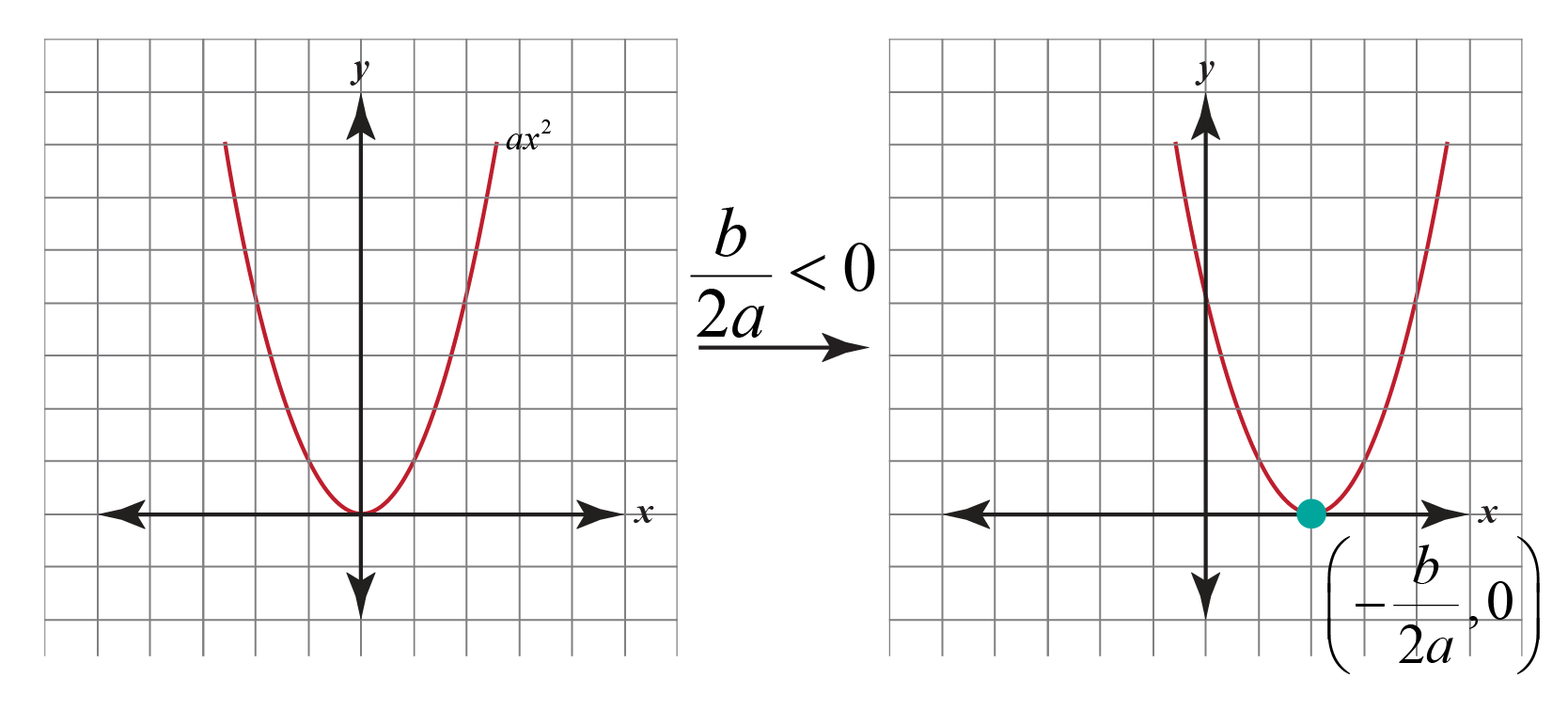Step-3: $$a{\left( {x + \dfrac{b}{{2a}}} \right)^2} \to a{\left( {x + \dfrac{b}{{2a}}} \right)^2} - \dfrac{D}{{4a}}$$: This transformation is a vertical shift of magnitude $$\left| {\dfrac{D}{{4a}}} \right|$$ units. The direction of the shift will be decided by the sign of $$\dfrac{D}{{4a}}$$. The final vertex of the parabola will be at $$\left( { - \dfrac{b}{{2a}},- \dfrac{D}{{4a}}} \right)$$. The following figure shows an example shift: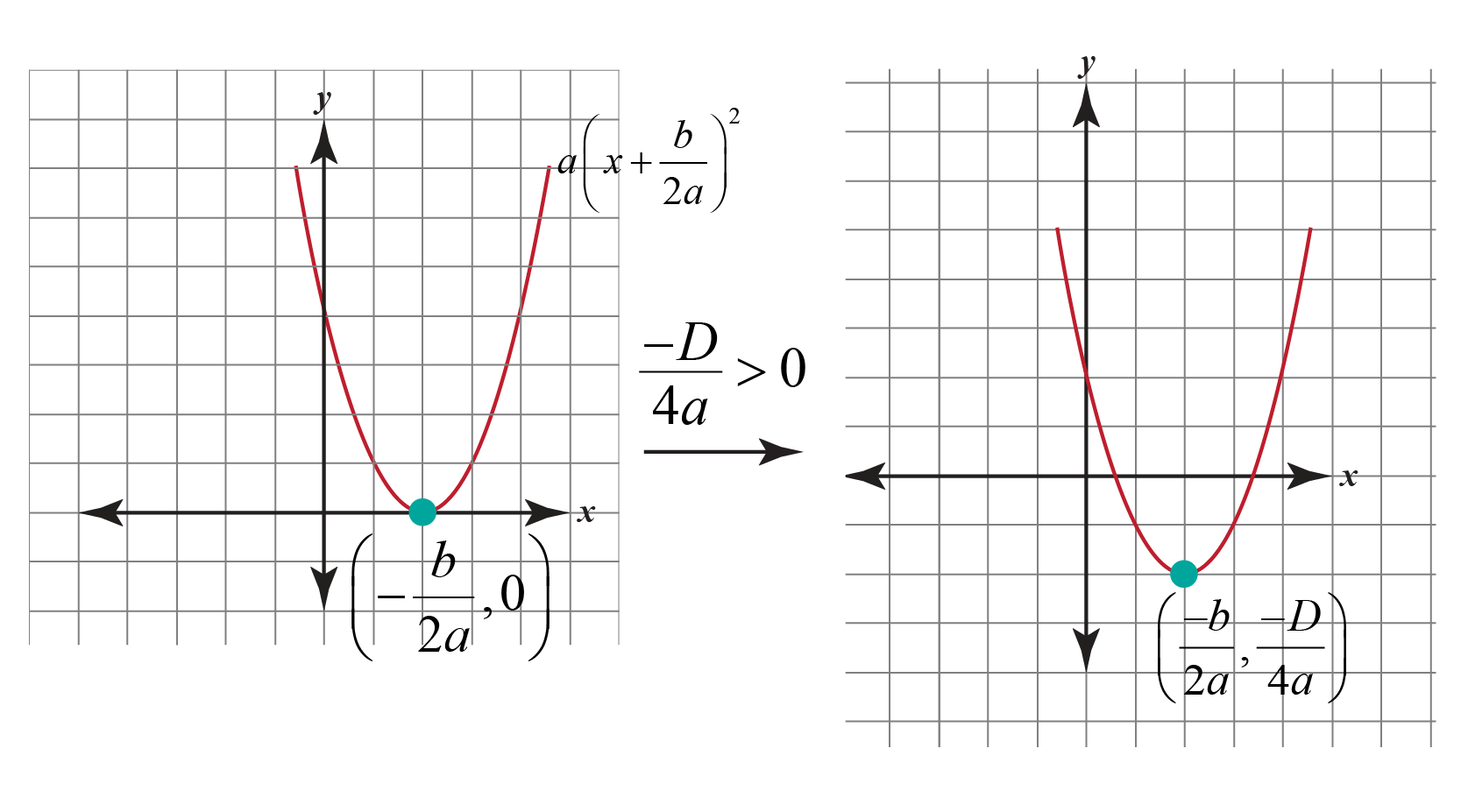Sometimes working with a hard quadratic function is difficult. But don’t worry!

Here are some tips for you to decide to which side the graph makes a frown or a smile.Tips and Tricks
 Let the vertex form of quadratic function be $$f(x)=a(x-h)^{2}+k$$. If $$a>0$$, then the parabola opens upward. If $$a<0$$, then the parabola opens downward.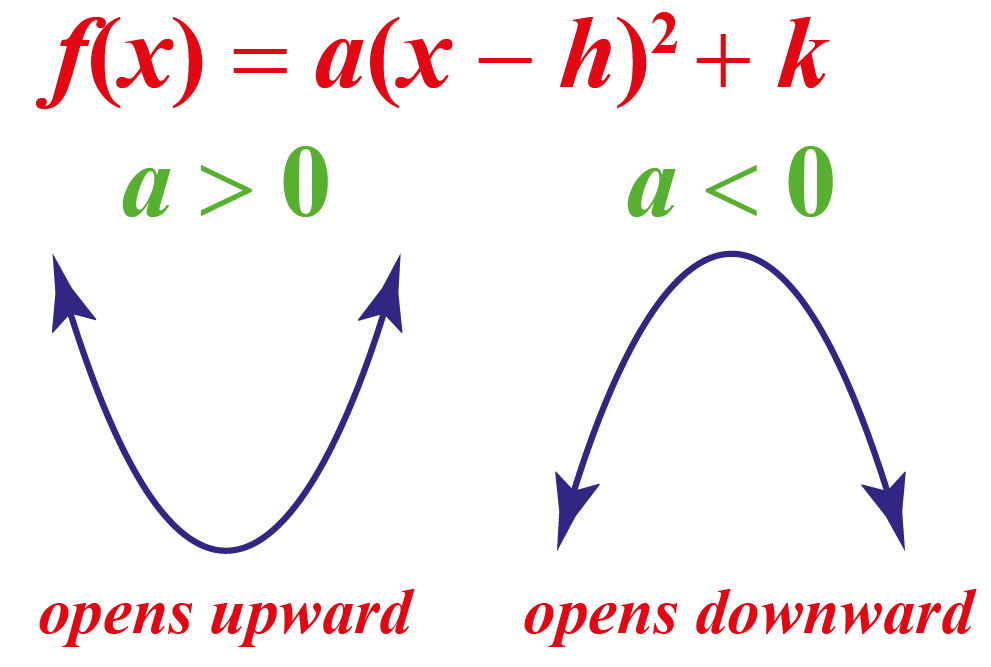## Quadratic Function Grapher

This is how the quadratic function is represented on a graph.

This curve is called a parabola.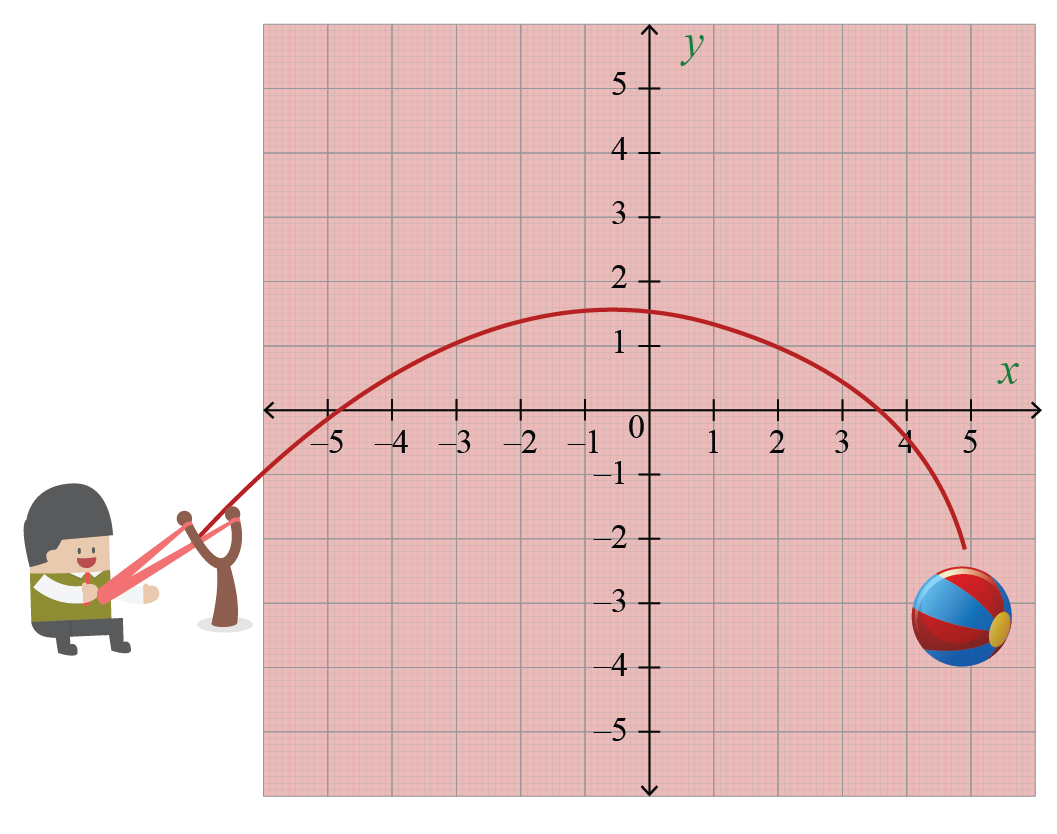Now let’s explore some quadratic equations on a graph using the quadratic function graph.

Drag the values of $$a$$ to check for the variations of the upward parabola and downward parabola.

Dragging the values of $$b$$ will move the curve either right or left.

Dragging the values of $$c$$ will move the curve either up or down.

Download Functions Worksheets
Functions
grade 10 | Answers Set 1
Functions
grade 10 | Questions Set 2
Functions
grade 10 | Answers Set 2
Functions
grade 10 | Questions Set 1
More Important Topics
Numbers
Algebra
Geometry
Measurement
Money
Data
Trigonometry
Calculus